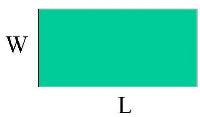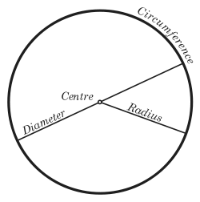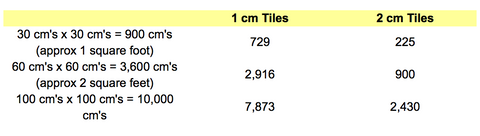0

# How Many Mosaic Tiles Do I Need for My Project?

March 17, 2015

When starting a project it's important to estimate how many tiles or tesserae you will need to complete the project. It's very frustrating running out of materials, having to wait to finish your project or finding out that the colour of the batch has changed since you made the initial purchase.

The amount of tiles you need will be affected by a few factors:
• The amount of grout you will use. Generally, the smaller the tiles the more grout you will use and vice versa, the larger the tiles the less grout.
• Wastage from badly cut or shattered tiles and the cutting of irregular shapes

Steps to Determining the Amount of Materials you need:

1. Measure the Size of your Surface

Get your tape measure or ruler out and measure the size of the surface that you are planning to mosaic. The area of the base can be calculated using the following formulas:

Rectangle or square area = length x widthTriangle area = 1/2 x width x length2. Use this quick look up guide to work out how many tiles you'll needNote: No wastage has been factored into this calculation so don't forget to add an allowance for additional tiles. This will be affected by how much cutting you are planning to do and your level of expertise.

3. A rough formula for calculating the amount of tiles yourself

Example:

Square base 50 cm's x 20 cm's = 1,000 cm's
2 cm tile surface area = 2 cm's x 2 cm's = 4 cm's square

How many tiles? 1000 / 4 = 250 tiles

4. Estimating irregular shapes

Rather than using complicated mathematical equations to determine the number of tiles for irregular shapes, you can estimate the number of tiles based on visualisation. For instance, visualise your oval shape as a circle or a hexagon as a smaller square to roughtly estimate the number of tiles.

You can also break complicated shapes up into smaller shapes or use this technique to roughly estimate the quantity of each colour.

5. Always buy more tiles (tesserae) materials than you need

It is always advisable to overestimate the amount of tiles or tesserae for the project rather than underestimate.